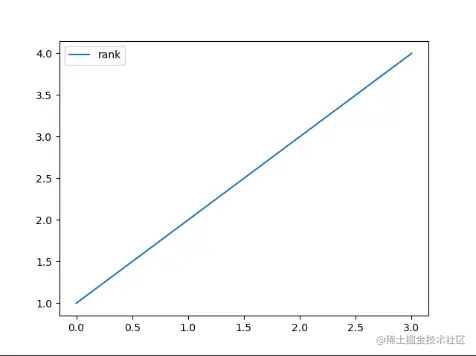# [pandas learning notes 02] - advanced usage of data processing

2022-02-01 19:12:07

author ： Illusory good

source ： Hang Seng LIGHT Cloud community

Pandas It's a Python Software library , It provides a large number of functions and methods that enable us to process data quickly and easily . This article will mainly introduce Pandas Practical data processing operations .

Series articles ：

【Pandas Learning notes 01】 Powerful tool set for analyzing structured data

【Pandas Learning notes 02】- Practical operations for processing data

## summary

Pandas Is based on NumPy Constructed library , In terms of data processing, it can be understood as NumPy Enhanced Edition , meanwhile Pandas It is also an open source project . It is used for data mining and data analysis , It also provides data cleaning function .

In this paper , This paper mainly introduces Pandas In data processing High-order usage , Include ： Data consolidation 、 Grouping and splitting . If you have studied database SQL grammar , This article will be very fast to understand .

## Data merging

#### Data preparation

So let's define a `DataFrame` Data sets ：

``````import pandas as pd
​
df_a = pd.DataFrame(columns=['name', 'rank'], data=[['C', 1], ['java', 2], ['python', 3], ['golang', 4]])
df_b = pd.DataFrame(columns=['name', 'year'], data=[['java', 2020], ['python', 2021], ['golang', 2022]])
Copy code ``````

adopt `merge()` The method can be right DataFrame Data sets are merged , Through internal connections 、 External connection 、 Left connection 、 Right connection, etc , The following example ：

merge The default method is to take the intersection of inner connections , adopt `how` Specify connection type ,`on` Specify the connection field

``````#  By designation  columns  Medium  name  Internal connection
df_tmp = pd.merge(df_a, df_b, on='name', how='outer')
print(df_tmp)
​
# ======== Print ========
name  rank  year
0    java     2  2020
1  python     3  2021
2  golang     4  2022
Copy code ``````
``````#  By designation  columns  Medium  name  Left connection
df_tmp = pd.merge(df_a, df_b, on='name', how='left')
print(df_tmp)
​
# ======== Print ========
name  rank    year
0       C     1     NaN
1    java     2  2020.0
2  python     3  2021.0
3  golang     4  2022.0
Copy code ``````
``````#  By designation  columns  Medium  name  The right connection
df_tmp = pd.merge(df_a, df_b, on='name', how='right')
print(df_tmp)
​
# ======== Print ========
name  rank  year
0    java     2  2020
1  python     3  2021
2  golang     4  2022
Copy code ``````
``````#  If you combine two  DataFrame  Excluding public  columns , You can specify matching fields directly
df_c = pd.DataFrame(columns=['name1', 'year'], data=[['java', 2020], ['python1', 2021], ['golang1', 2022]])
df_tmp = pd.merge(df_a, df_c, left_on='name', right_on='name1')
print(df_tmp)
​
# ======== Print ========
name  rank name1  year
0  java     2  java  2020
Copy code ``````

## The data packet

#### Data preparation

So let's define a `DataFrame` Data sets ：

``````import pandas as pd
​
df_a = pd.DataFrame(columns=['name', 'nums'], data=[['python', 1], ['java', 2], ['python', 3], ['java', 4]])
Copy code ``````

adopt `group()` The method can be right DataFrame Data sets are grouped , After grouping, it can be summed 、 Take the average, etc , The following example ：

``````#  Gets the number of each data in the grouped dataset
df_tmp = df_a.groupby('name').size()
print(df_tmp)
​
# ======== Print ========
name
java      2
python    2
dtype: int64
Copy code ``````
``````#  The grouped data set , according to  nums  Field to sum
df_tmp = df_a.groupby('name')['nums'].sum()
print(df_tmp)
​
# ======== Print ========
name
java      6
python    4
Name: nums, dtype: int64
Copy code ``````
``````#  Gets the size of the grouped dataset
df_tmp = df_a.groupby('name').size()
print(df_tmp)
​
# ======== Print ========
name
java      3
python    2
Name: nums, dtype: int64
Copy code ``````

## Data splitting

#### Data preparation

So let's define a `DataFrame` Data sets ：

``````import pandas as pd
​
df_a = pd.DataFrame(columns=['name', 'rank'], data=[['C_no1', 1], ['java_no2', 2], ['python_no3', 3], ['golang', 4]])
Copy code ``````

adopt `split()` The method can be right DataFrame Split a column of data in the dataset , The following example ：

``````#  Data splitting , Yes  columns  The data of a column in matches a symbol ,expand： by True The results can be directly converted into DataFrame
df_tmp = df_a['name'].str.split('_', 1, expand=True)
print(df_tmp)
​
# ======== Print ========
0     1
0       C   no1
1    java   no2
2  python   no3
3  golang  None
Copy code ``````
``````#  Data splitting , Merge the split data with the original data again
df_tmp = pd.merge(df_a, df_a['name'].str.split('_', 1, expand=True), how='left', left_index=True, right_index=True)
print(df_tmp)
​
# ======== Print ========
name  rank       0     1
0       C_no1     1       C   no1
1    java_no2     2    java   no2
2  python_no3     3  python   no3
3      golang     4  golang  None
Copy code ``````

## Data visualization

In the use of Pandas In the process of processing data , In order to more intuitively show the linear relationship of data , We can introduce `matplotlib` The library turns our data into related graphics

``````# plot()  Method to generate the corresponding linear graph
df_a = pd.DataFrame(columns=['name', 'rank'], data=[['C_no1', 1], ['java_no2', 2], ['python_no3', 3], ['golang', 4]])
df_a.plot()
Copy code ``````## summary

This paper mainly introduces Pandas High level operations of toolset , The operating principle is the same as that in the database SQL It's the same thing , It can help us solve daily data analysis and processing .